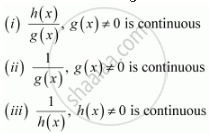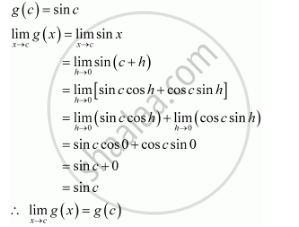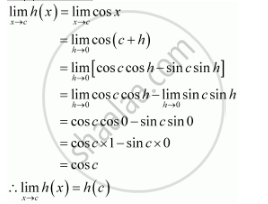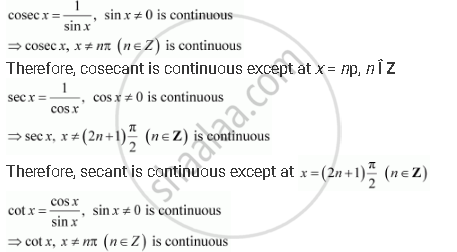Share

# Discuss the Continuity of the Cosine, Cosecant, Secant and Cotangent Functions, - CBSE (Commerce) Class 12 - Mathematics

#### Question

Discuss the continuity of the cosine, cosecant, secant and cotangent functions,

#### Solution

It is known that if and are two continuous functions, thenIt has to be proved first that g (x) = sin and h (x) = cos x are continuous functions.

Let (x) = sin x

It is evident that g (x) = sin x is defined for every real number.

Let be a real number. Put x = c + h

If x → c, then h → 0Therefore, g is a continuous function.

Let h (x) = cos x

It is evident that h (x) = cos x is defined for every real number.

Let be a real number. Put x = c + h

If x ® c, then h ® 0

(c) = cos cTherefore, h (x) = cos x is continuous function.

It can be concluded that,Therefore, cotangent is continuous except at np, Î Z

Is there an error in this question or solution?

#### APPEARS IN

NCERT Solution for Mathematics Textbook for Class 12 (2018 to Current)
Chapter 5: Continuity and Differentiability
Q: 22 | Page no. 160

#### Video TutorialsVIEW ALL 

Solution Discuss the Continuity of the Cosine, Cosecant, Secant and Cotangent Functions, Concept: Algebra of Continuous Functions.
S